### Home > CALC > Chapter 3 > Lesson 3.2.3 > Problem3-81

3-81.
1. Evaluate each limit. If the limit does not exist, say so but also state if y is approaching positive or negative infinity. Homework Help ✎

1.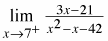2.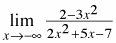3.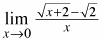4.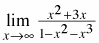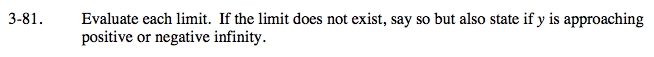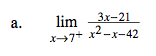Factor. The limit might exist.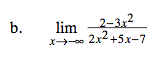As with all limits to ∞ or −∞, we are looking for horizontal asymptotes, if there are any. So only consider the terms with the highest powers. And do not forget about their coefficients. Also, notice that this limit is approaching −∞.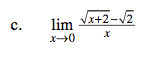You should recognize that this is a definition of a derivative?Whose form is it: Hana, Anah, Hanah or Ana?

There is no h, so this is Ana's definition:

$\lim_{h\rightarrow a}\frac{f(x)-f(a)}{x-a}$

If you can figure out what f(x) and a are, then you could use the Power Rule shortcut.

$f(x)=\sqrt{X+2}\text { and }a=0.\text{ This can be verified}$

$\text{ because }\sqrt{0+2}=\sqrt{2}$

$\text{Since }f(x)=\sqrt{x+2}=(x+2)^{\frac{1}{2}}$

$f'(x)=\frac{1}{2}(x+2)^{-\frac{1}{2}}=\frac{1}{2\sqrt{x+2}}$

$f'(0)=\frac{1}{2\sqrt{0+2}}=\frac{1}{2\sqrt{2}}$Refer to the hint in part (b). Notice that, in this case, the denominator has a larger power than the numerator.